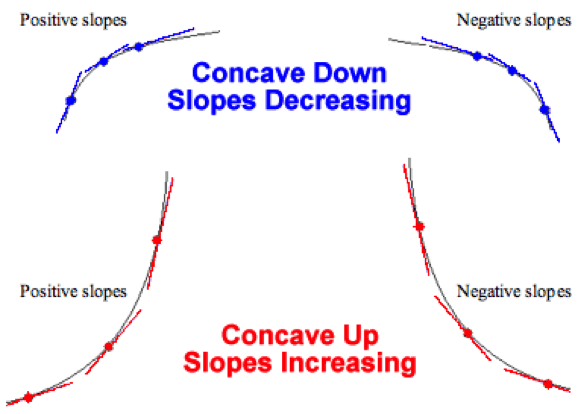Give us feedbackX

# Second Derivative Calculator

23 Sep 2015Homework Help Online

What is Second Derivative Calculator?

The second derivative calculator is a tool which helps to find the derivative of the derivative of function f. This online calculator makes the calculations easy and enjoyable. Also the problems can be solved instantly. It is used to calculate the second derivative of a function (f). Differentiation is a method to calculate the rate of change (or the slope at a point on the graph).

Examples:

1. Find the second derivative of the function: 3x2?
Step 1:  Given function: 3x2
F′ (x) = ddx (3x2) => 3 ddx (x2)
F′ (x) = 3 (2 x) => 6x
Step 2:  F′′ (x) = ddx (6x)
F′′ (x) = 6 ddx (x)
F′′ (x) = 6(1)
F′′ (x) = 6
Answer:  Second derivative = 6
2. Find the second derivative of the function: 12 x4?
Step 1:  Given function: 12x4
F′ (x) = ddx (12x4) => 12 ddx (x4)
F′ (x) = 12 (4 x3) => 48x3
Step 2:
F′′ (x) = ddx (48x3)
F′′ (x) = 48 ddx (x3)
F′′ (x) = 48(3x2)
F′′ (x) = 144 x2
Answer:  Second derivative = 144 x2

Uses of Second Derivative Calculator:

• The Second derivative calculator shows the step by step differentiation. Also, it helps in computing first, second, third ...sixth derivatives
• It also helps in differentiating functions with many variables or partial derivatives
• It calculates roots / zeros as well
• By using the second derivative of a function, it is also practicable to find the relative maxima and minima. For this, calculate the first derivative of the function and locate its critical points. After the first derivative, calculate the second derivative of the function. Then plug the critical points found in the second derivative. The results show whether it’s a relative maximum or minimum
• If the result is Positive = Relative minimum (the graph is concave up at that point)
• If the result is Negative = Relative maximum (the graph is concave down at that point)
• If the second derivative is 0 at a critical point, then the test has failed and you have to use the first derivative test to find out if that point is maximum or minimum

Note:

• Maximum or maxima (plural) is the high point
• Minimum or minima (plural) is the low point

Generally, the word extremum (plural extrema) is used for maximum or minimum, also local maximum or minimum are used when there are possibly higher or lower points away but not close by.

The schematic diagrams, graphs and plots are helpful in understanding the functions in an interactive way.The concavity of the graph of f is measured by the second derivative of a function f. Concave up refers that the second derivative is positive which is sometimes called convex also. It means the tangent line will lie below the graph of the function. Concave down refers that the second derivative is negative, also called simply concave and the tangent line will lie above the graph of the function.

https://www.symbolab.com/solver/second-derivative-calculator

http://www.derivative-calculator.net/

http://calculator.tutorvista.com/math/580/second-derivative-calculator.html

http://www.emathhelp.net/calculators/calculus-1/derivative-calculator/

http://community.wolfram.com/groups/-/m/t/283665

http://www.analyzemath.com/calculus/Differentiation/find_derivative_function.html

http://www.rasmus.is/uk/t/F/Su41k05.htm

https://en.wikipedia.org/wiki/Second_derivative

Related Posts

Testimonials

• Jason L.

I have a lot of questions that need to be answered. While searching online, I found the site of StudyFAQ and it is all worth it. The tutor is very kind and I’ve got everything I needed. Thank you so much.

• Garry T.

Tutor did a great job. The process was very fast and efficient. I didn’t know that there is a quality and reliable tutoring service provider online. I can now start with my assignment.

• Margret O.

I was really having a hard time finding an expert who can help me with my topic. Thanks to StudyFAQ.com, I can start working on my assignment now.